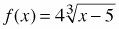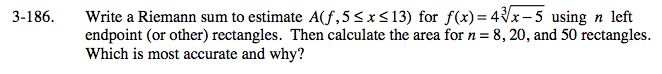### Home > CALC > Chapter 3 > Lesson 3.4.4 > Problem3-186

3-186.

Write a Riemann sum to estimate A(f, 5 < x < 13) forusing n left endpoint (or other) rectangles. Then calculate the area for n = 8, 20, and 50 rectangles. Which is most accurate and why? Homework Help ✎Refer to hints in problem 3-177 for further guidance about computing a left-endpoint Riemann sum.

Without a calculator, it is more time consuming to compute a sum with 50 terms than with 8 terms. What is the benefit of using 50 terms?